## Monday, March 4, 2013

### Output Inductor Calculation for SMPS Converters using the Forward, Push-Pull, Half-Bridge and Full-Bridge Topologies

An output inductor is found at the output of every forward-mode converter. Converters utilizing the forward, push-pull, half-bridge and full-bridge topologies are all forward-mode converters. So, calculation of the output inductance follows the same methodology for all four of these popular topologies. The purpose of the output inductor is to store energy for the load during the time each switching cycle when the power switches (BJTs, MOSFETs or IGBTs) are turned off. The electrical function of the output inductor is to integrate the rectangular switching pulses (pulse width modulated signals with varying duty cycle) into DC. The capacitor following the inductor smooths the DC into clean DC.

The design of the output inductor is quite simple. Usually, a self-gapped toroid core is used. Gapped ferrite cores (the ones used for ferrite transformers, eg ETD39) can also be used with no difficulties.

The formula for calculating the output inductance is:

• Vin(max) is the highest peak voltage following the output rectifier of that particular output.
• Vout is the output voltage.
• Toff(est) is the estimated on time of power switches at the highest input voltage.
• Iout(min) is the lightest expected load current for that output.

Calculated from the above equation is Lmin – the minimum required inductance, below which the core will empty of flux at the minimum rated load current for that output. You may want to design a circuit that allows for operation with no load. Obviously, you can’t substitute zero for Iout(min) as that will result in an Lmin value of infinity. And well, that really isn’t possible, is it?

So, what you should do is that you should select a minimum allowable current. Use a resistor load at the output of the power supply so that when you have no other loads, this resistor load provides the minimum load. Iout(min) should be large enough that Lmin isn’t too large; it should also not be too large that you have too high power losses, and thus too low efficiency, due to the power dissipation in the output resistor. Sometimes, this resistor is referred to as a dummy load – its only purpose is to provide the minimum load when there is no other load at the output of the converter / power supply.

Now that we know the minimum required inductance, we need to know the number of turns to wind on our core.

From the datasheet of the core, you can find the AL value. This indicates the inductance per turns squared:

L is the inductance and N is the number of turns. Making N the subject:
So, that’s the formula used to calculate the number of turns once we know the required inductance.

In some cases you might not know the AL value. You may not know the part number of the core you have and thus cannot look for the datasheet. Whatever the reason, you can experimentally determine the AL value.

Wind a few turns and measure the inductance. Then, measure the inductance for a different number of turns. Repeat for different numbers of turns. So, measure the inductance for, for example, 5, 10, 20, 40 turns and then for each, calculate the AL value. Take the mean AL value. Another thing you could do is, you could draw a graph of L against N2. The gradient of the best fit line would be the AL value. You could also mathematically calculate the gradient of the “regression line”. Use whichever method you find simplest.

Now let’s look at an example to clear up what you’ve read till now.

Let’s say that our converter is a half-bridge converter. For ferrite transformer calculation in offline half-bridge converter, refer to this tutorial:

Back to our example.

The input voltage to the converter will vary from 150VAC (212VDC) to 250VAC (354VDC). Output voltage of the converter is 14VDC. The switching frequency is 50kHz.

Transformer primary: 26 turns
Transformer secondary: 4+4 turns

The formula for calculating the minimum required inductance is:

We need to calculate the output voltage at the transformer secondary at 354VDC input, which is our maximum input voltage.

We’ll assume that the voltage drop due to the rectifier diode is 1V. Thus the average output voltage at the transformer secondary is 15V. Transformer turns ratio (primary : secondary) = 26:4 = 6.5

So, when average secondary voltage equals 15V, average voltage across transformer primary is 6.5 * 15V = 97.5V. If duty cycle was 100%, voltage across transformer primary would be 177V (half the DC bus voltage – think half-bridge topology). So, the duty cycle is (97.5/177)*100% = 55%.

Average output voltage at transformer secondary is 15V with a duty cycle of 55%. Thus, peak output voltage is 15V/0.55 = 27.3V, after which we have assumed a diode drop of 1V. So, Vin(max) is 26.3V.

At maximum input voltage, duty cycle will be lowest. This is when the off time will be the highest.

We have calculated a duty cycle value of 55% - this is the lowest duty cycle value. As switching frequency is 50kHz, time period is 20µs. The off time is 0.45 * 20µs = 9µs. That is our Toff(est).

Let’s say that the minimum load will draw 500mA current. With a 14V output and 500mA current, the power dissipated in the output resistor will be:

P = VI = 14 * 0.5 W = 7W

That is a lot of power! If it’s acceptable, go ahead and use a 500mA minimum load. If you choose to bring the minimum load down to 250mA, you bring down power dissipation (above) to 3.5W.

So we now have all required parameters. Let’s plug them into the formula.

This is the minimum required inductance. You should use an inductance larger than the minimum value calculated, since, well you calculated the minimum required inductance.

Let’s say we’ll use an inductance of 450µH. Let’s say that we’ve selected a toroid core with an AL value of 64nH per turn squared.

Firstly, the required inductance is 316µH which is equal to 316000nH.

Thus the required number of turns is:

You can use either 70 or 71 turns. This is for 316µH.

For 450µH:

Round this up to 84 turns.

There we have it. You can use this simple method to calculate the required output inductance for a converter that uses the forward, push-pull, half-bridge or full-bridge topology. It’s simple and I hope I’ve been able to make you understand clearly. Let me know your comments and feedback!

1.Dear Tahmid
Its really very useful I was searching for this materials you provide me hint thank you. I usually read your blog in one of your blog you had mention that you was developing SMPS design tool can you say when you are going to release.

Regards
Veera..!

1.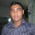It's great that you found it helpful. Thanks!

I'm not sure when I'm going to release it. I haven't been working on it recently. I've been very busy, and my free time largely goes by writing stuff for this blog.

I'm sure if not the software, at least the articles themselves will help you make the necessary calculation!

Regards,
Tahmid.

2.Good work Tahmid, i hope u will be a lecturer or an instructor one day. you give it out as simple as it can be. well done.

1.Regards,
Tahmid.

3.Tahmid, i looked over your calculation for number of turns for an inductor.
“ Let’s say we’ll use an inductance of 450µH. Let’s say that we’ve selected a toroid core with an AL value of 64nH per turn squared.

The required inductance is 316µH which is equal to 316000nH.”

Turns = square root of (316000nH / 64nH per turn) = 70.3 turns.

Now that i see nH over nH in an example it makes perfect sense, The nH cancels out and you have turns left. It did not dawn on me you should just convert uH to nH for the equation.

In the past i needed to calculate turns for mH and i looked a long time to find a formula that had a constant in it so i could use mH for the inductor and nH for AL. After reading your post i converted my .1mH to 100,000nH and divided by my AL of 405nH per turn and got the same 15.71 turns i got with the other equation i found. Once again good work, you are very good at explaining things in simple terms that anyone can understand.

4.Tahmid, That was a very good write up on inductor design for forward converters. It has more information than i have seen anyware.

I slowly worked thru your method for calculating inductance and then i re-read all my literature on calculating inductors. Most methods were based on your formula with slight variations. Your comprehensive write up helped me understand the other variations of the formula.

One of the differences in the formulas is what to use for I min. G Chryssis says .25Imax, M Brown does not say but used 10% in one example. Schmidt-walter says "L is chosen so that ΔIL=0,4 x Iout for >Vin_max". Pressman derivation of his formula says he uses 10% of I max.

If I use 10% Imax in your formula “1.78A” (250W / 14vdc = 17.8A) then i get a L of 44.4uH.

I used your numbers for the half bridge converter you designed and entered them in the other formulas.

http://schmidt-walter.eit.h-da.de/sm...gw_smps_e.html

gave an inductance of 9.32uH.

G Chryssis book said 16uH at .25Imax. But at 10% was 39uH

Tahmid method at 10% says 44.4uH

Pressman’s book said 47uH. Based on 10%

M Brown book said 57uH. Based on 10%

If we throw out schmidt-walter, you ended up approximately in the middle of the other calculations, so i would say your write up has to be correct. M Brown’s value is a little high because he does something strange. He says that “Toff(est)” means “ON time” so that explains why his value is unexpectedly high.

One last thing on Imin for these calculations. Imin does not mean that the power supply must not go below that current. It only means that the output goes into discontinuous mode after Imin and you loose the benefit of the inductor. If you have a regulated supply it will stay regulated. So you do not need to place a load resistor on the output to make Imin.

5.Hi Tahmid,

One thing I can't find addressed in your explanation is where the "1.4" figure comes from. I'm guessing it's related to the ripple current in the inductor, but could you elaborate on this for us here in the cheap seats? :)

6.Hi Tahmid,
Thanks for supporting designers,

we r working on 1KW inverter with below specs

12v-350v dc-dc converter with pushpull topology

e65 core ferrite

full bridge topology with igbt for ac-dc inversion

we r planning to provide the isolated feedback with a transformer

transformer specs are input will be from e65 core transformer and output must be scaled down to 5v dc to connect to sg3525.

plz provide us the details of the transforme like core to be used and no tunrs

7.Dear Tahmid

can you please explain what is this "unsigned char FlagReg; and sbit Direction at FlagReg.B0;"

Regards
Veera

1."Direction" is a flag that I used to determine the direction of the bridge - to determine which MOSFETs will be on, when. Now, since it's a binary flag - taking only zero or one - it must be part of a register. So, I defined an 8-bit register FlagReg, of which bit 0 is the bit "Direction" which I use as a flag.

Regards,
Tahmid.

8.Hi, Tahmid. It's very interesting subject. I have a little question as I am a beginner in SMPS and very curious about it, here is my question, What would happen if we use output inductor that has smaller output inductor? For example from your above calculation, instead of using 84 turns, what would happen if we use only 40 turns? Is it going to heat up the switching transistor? Or maybe limiting the output current of the SMPS? Or what?

Your explanation would be very helpful for me to make better understanding on this subject, Thanks

1.Hello Tahmid, what is the different in core design method for dc-dc output filter inductor and dc-ac output low pass filter inductor for spwm inverters.
Regards

2.One obvious thing that would happen by reducing the turns is that it will increase the flux density. Now, if the flux density is increased too much, the core can saturate and there will be a short-circuit and you damage the circuit.

9.Hi Thamid, I found very helpful and interesting all your posts. Thanks to your explanation I have designed and built succesfully a push pull 300W dc/dc converter 12 V to 300V for use in geological survey. I am now at the calculus of the output inductor. Using the formula to determine Lmin if Vin(max) is less than Vout brings to a negative voltage value. How to overcome this problem?
Regards

10.hello Tahmid

could give an example of calculation of a half-bridge smps with the following data:

220Vac 310vdc
pri turns 32
sec 3 +3 turns
frequency 27kHz
secondary voltage 15v
70A output I
inductor with Al 100nH/esp ²

very good blog
grateful

11.great blog, thx

12.Tahmid, I have one quick simple question.

Q: in fly-back converters design, the transformer is connected to a DC voltage input but it only works with AC because of variable magnetic field which DC voltage can not provide. How did they achieve the induction of secondary voltage by the primary DC voltage?

I know there is a MOSFET switch and it disconnects the DC voltage in a very short time (before di/dt becomes 0) but is it the answer?

1.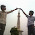yes...it is the answer...the frequence is too high ( time period is small )...which makes the dc voltage a square wave...so now amplitude is not constant..it is varying w.r.t time...and hence voltage is induced in secondary winding.

13.pls tahmid can u tell me how to identify an ETD44 using a metre ruler

14.congratulations on the blog, has been very useful. I have a doubt in the calculation of capacitors for the H half bridge.
Will could help me ?
greetings
José Campos

15.Dear Tahmid i am new in this block i need your help i am doing a battery charger with halp brige topology its a 48v@12amp cahrger with pwm ic 33525 the output is able to deliverd 12amps but my probleum is coming when i am puting in cabnet (box alumunium) mens when i am going to coverd it than current going down from 12amps to 9 amps i am vary confused why its happen i am new in power electronics field Please help me

16.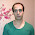This comment has been removed by the author.

17.Interesting blog. It takes some time to understand but I must say you have described in very user friendly way which will be helpful for all to understand. Thanks for sharing.

18.sir
i want to design a high power dc supply and i intend to use toroid transformer,from 230 v ac input to 1000-1500 v dc output and about 200 mA current.i intend to rectify and switch the 230 v to high freq like 20 khz.is this feasible.want your help

19.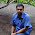hi,
From where the constant value 1.4 comes to the denominator of Lmin equation.

20.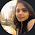Hello Tahmid, How can we construct a LC filter with out using a transformer. I tried using LCR filter in between the full H bridge, but I'm not getting a clean sine wave. I need some help regards to that.
Thanks.

21.I found your this post while searching for information about blog-related research ... It's a good post .. keep posting and updating information. best-film-to-digital-converters

22.23.